# Variation on Connected Objects – Using Graphs

Reader Uri Pe'er found an old post from 2011 about shortest paths in binary images, and he asked this follow-up question (paraphrased):

How can I determine if there is a path from one row to another in a binary image? And can I constrain the direction of movement along the path?

Last week I showed how to tackle this problem using imfill. Today I'm going to use a graph.

In December 2015 I wrote about how to create graphs based on the connectivity of image pixels, and I submitted some functions to the File Exchange to make it easy.

One of those functions is binaryImageGraph. Let's try it using one of the same images from my last post.

bw1 = imread('https://blogs.mathworks.com/steve/files/path-shapes-1.png');
imshow(bw1)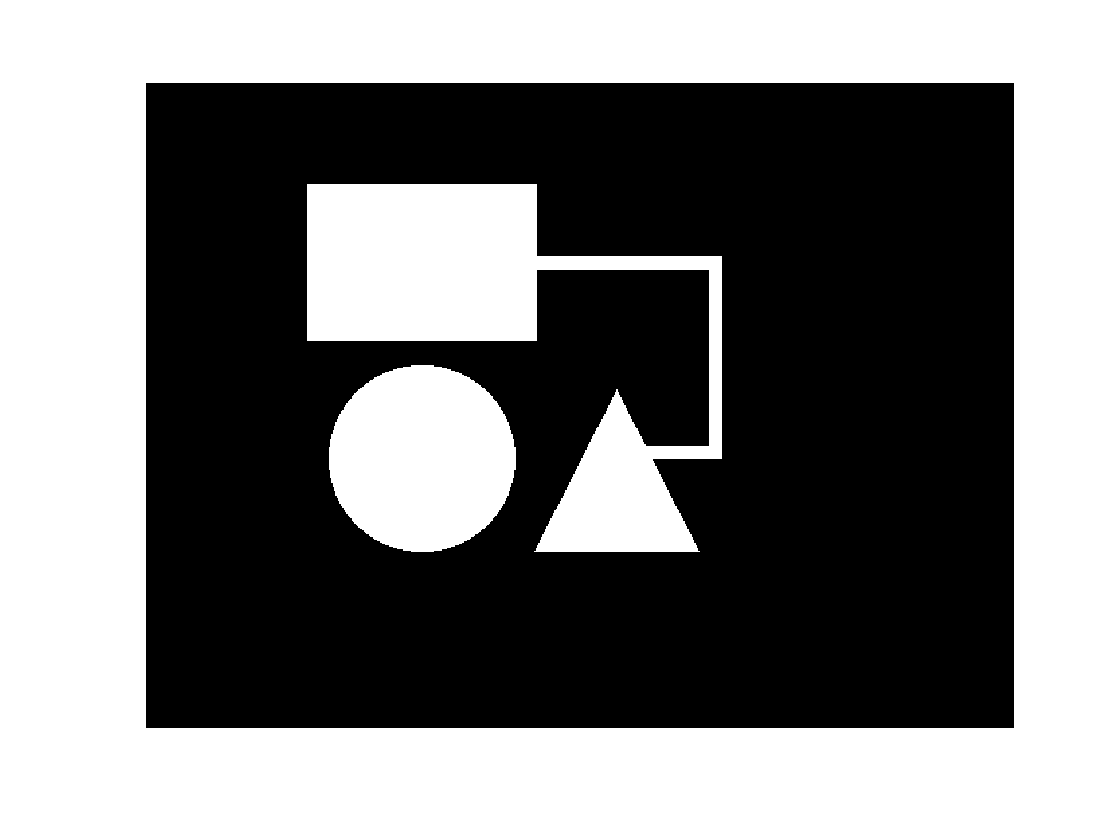g = binaryImageGraph(bw1)

g =

graph with properties:

Edges: [180444×2 table]
Nodes: [45880×3 table]



Each foreground pixel in the binary image corresponds to a graph node, so the total number of graph nodes is the same as the number of foreground pixels.

nnz(bw1)

ans =

45880



The function plotImageGraph (from the same File Exchange submission) can be used to visualize the image graph.

plotImageGraph(g)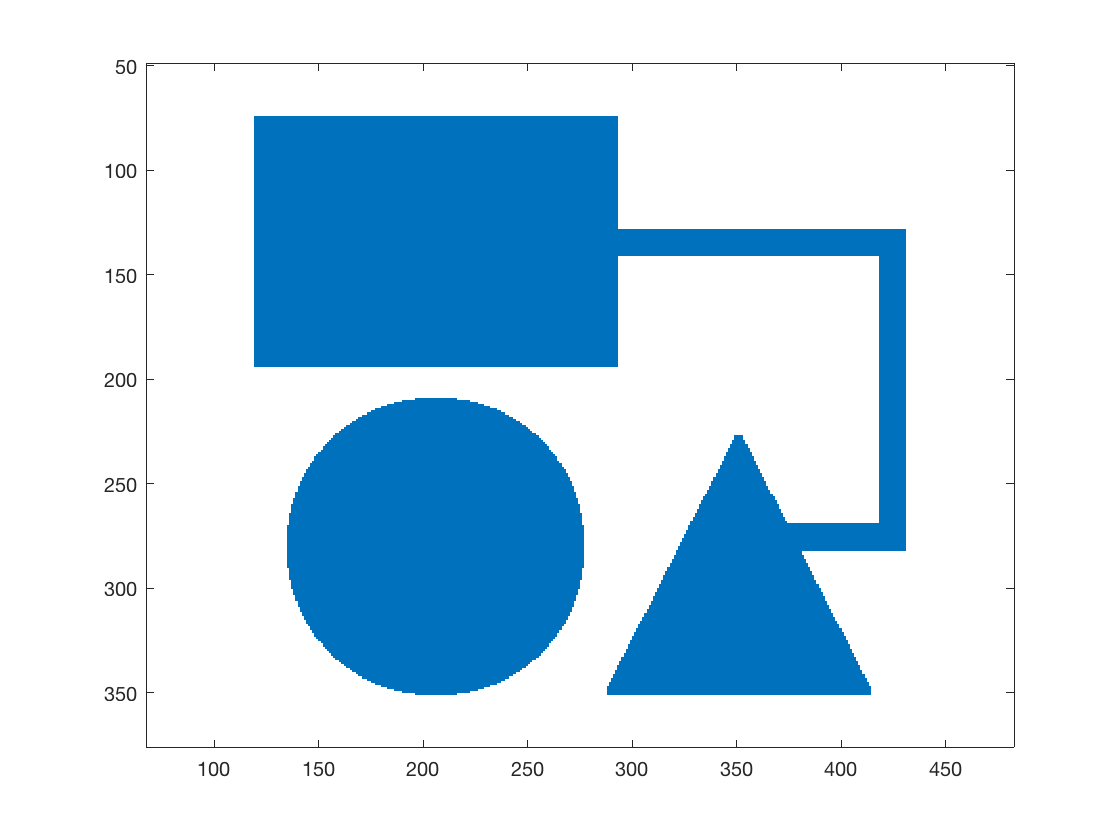Zoom in to see the graph details in a small portion of the image.

axis([275 300 125 140])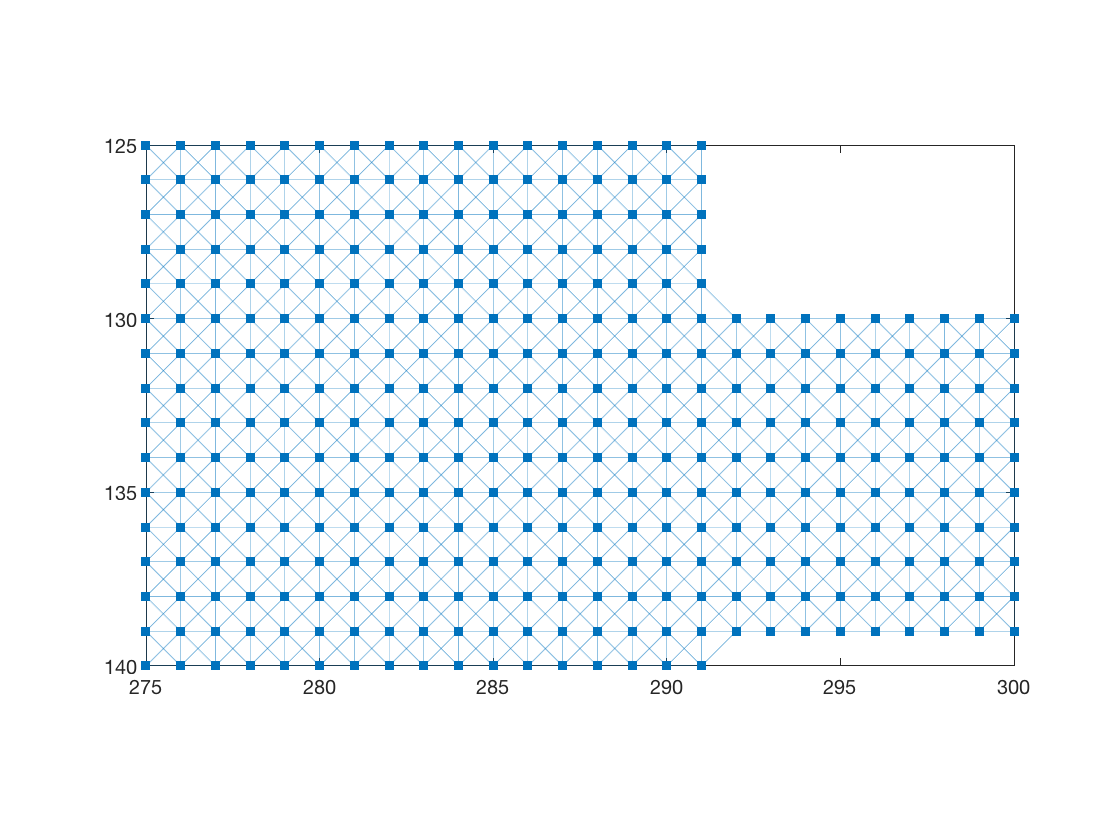Now let's figure out whether there are any graph paths connecting a row-100 pixels to a row-300 pixel. Note that binaryImageGraph stores the original foreground pixel locations in the graph's node table for purposes just like this. Here's what the node table looks like.

head(g.Nodes)

ans =

8×3 table

x     y     PixelIndex
___    __    __________

121    76    57676
121    77    57677
121    78    57678
121    79    57679
121    80    57680
121    81    57681
121    82    57682
121    83    57683


row_100_nodes = find(g.Nodes.y == 100);
row_300_nodes = find(g.Nodes.y == 300);


Next, use conncomp to find the connected components of the graph.

bins = conncomp(g);


For one of the graph nodes, bins(node) tells you which connected component that node belongs to.

unique(bins(row_100_nodes))

ans =

1



So the foreground pixels on row 100 all belong to the same connected component.

unique(bins(row_300_nodes))

ans =

1     2



The foreground pixels on row 300 belong to two different connected components. Since one of those the components is the same as for the row 100 pixels, that means there is path from row 100 to row 300.

Just like imfill, binaryImageGraph can take a custom connectivity matrix. We can use that to constrain the allowed paths. Let's try that with one of the other images I used last time.

bw3 = imread('https://blogs.mathworks.com/steve/files/path-shapes-3.png');
imshow(bw3)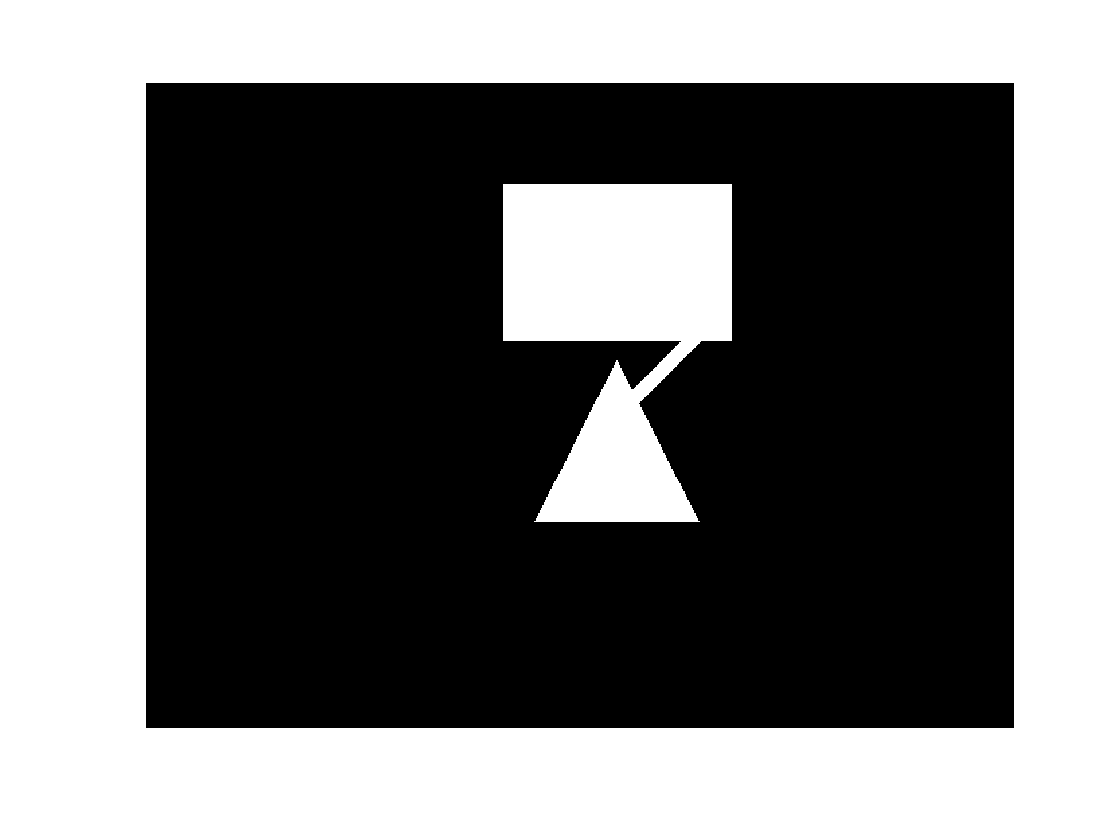conn = [
1 0 0
0 1 0
0 0 1 ];
g = binaryImageGraph(bw3,conn);
plotImageGraph(g)
axis([275 300 125 140])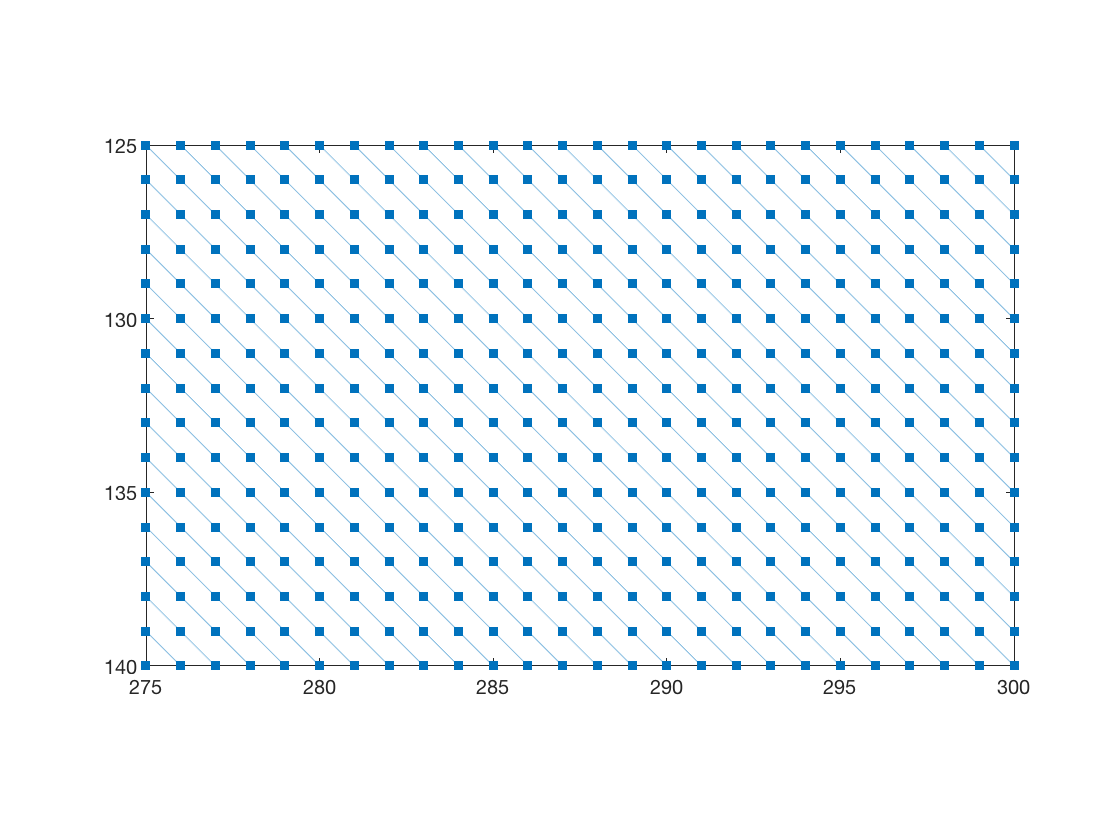Is there a constrained path from row 180 to row 250?

row_180_nodes = find(g.Nodes.y == 180);
row_250_nodes = find(g.Nodes.y == 250);
bins = conncomp(g);
~isempty(intersect(bins(row_180_nodes),bins(row_250_nodes)))

ans =

logical

0



No.

But what if we reverse the path constraint?

conn = [
0 0 1
0 1 0
1 0 0 ];
g = binaryImageGraph(bw3,conn);
plotImageGraph(g)
axis([275 300 125 140])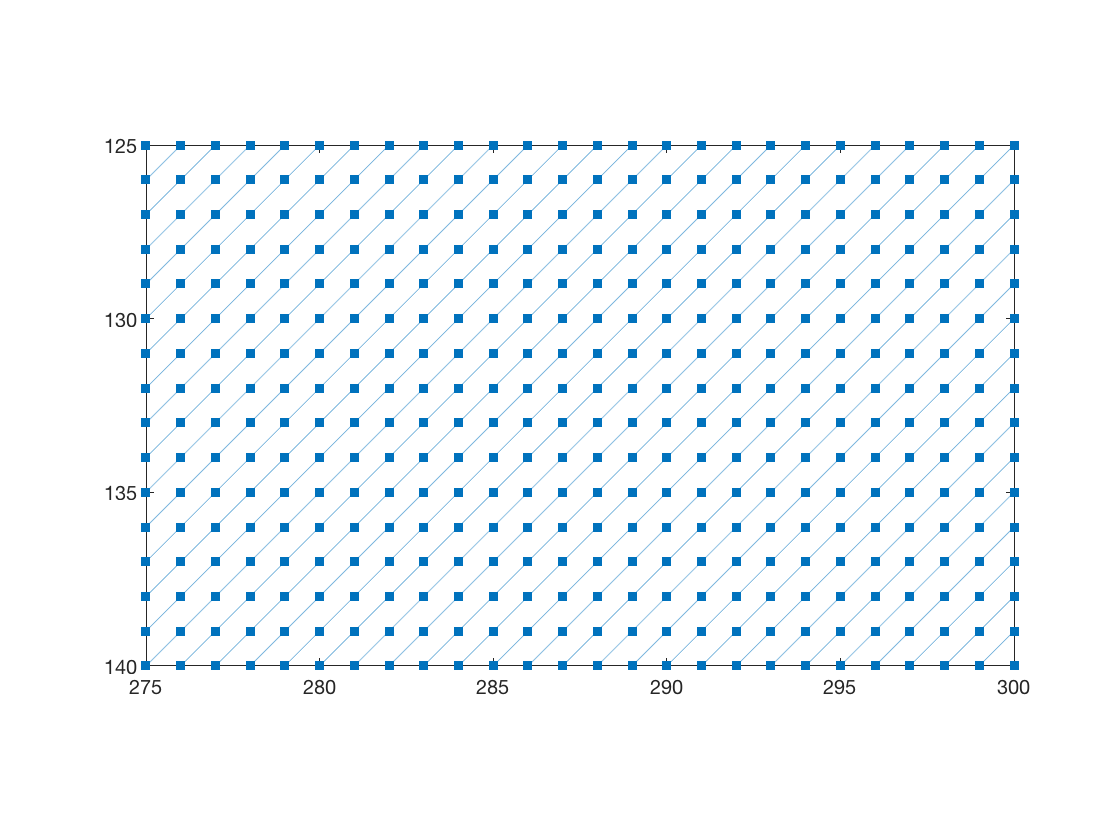row_180_nodes = find(g.Nodes.y == 180);
row_250_nodes = find(g.Nodes.y == 250);
bins = conncomp(g);
~isempty(intersect(bins(row_180_nodes),bins(row_250_nodes)))

ans =

logical

1



Yes, rows 180 and 250 are connected via a paths constrained to lie along the upper-right to lower-left diagonal.

Published with MATLAB® R2017a

|